# 25 and Level 3

25 is a composite number, and it is 5 squared. 25 = 1 x 25 or 5 x 5. Factors of 25: 1, 5, 25. Prime factorization: 25 = 5 x 5, which can also be written 25 = 5².

Since √25 = 5, a whole number, 25 is a perfect square.When 25 is a clue in the FIND THE FACTORS puzzles, write 5 in both the corresponding factor row and the factor column.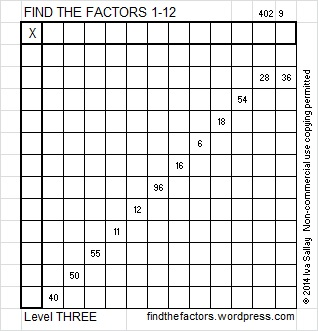Write the numbers 1-12 in the top row and again in the first column so that those numbers are the factors of the given clues. Use logic, not guessing and checking, to find its unique solution. Level 3 puzzles are designed to be solved starting from a row at the top of the puzzle with 2 clues. First find the common factor of those two clues that will allow you to write only numbers from 1 to 12 in the factor row at the top of the puzzle. Then factor row by row to the bottom of the puzzle making sure each number from 1 to 12 is written only once in both the factor row and the factor column. You will notice a rhythm for the answers as you work. Good luck!

This week’s puzzles are also available in an excel file here if you have a spreadsheet program on your computer. If you enable editing in excel, you can type your answers directly onto the puzzle, and you can also easily print the puzzles.

Here is the solution to last week’s level 3 puzzle:# 16 Silver Bells

16 is a composite number, and it is 4 squared. 16 = 1 x 16, 2 x 8, or 4 x 4. Factors of 16: 1, 2, 4, 8, 16. Prime factorization: 16 = 2 x 2 x 2 x 2, which can also be written 16 = 2⁴.Since √16 = 4, a whole number, 16 is a perfect square.

When 16 is a clue in the FIND THE FACTORS puzzles, use either 2 x 8 or 4 x 4. Only one of those sets of factors will work for any particular puzzle.

“Silver bells, silver bells.
It’s Christmas time in the city.”

Find the Factors is a type of logic puzzle. To solve one of the above puzzles, place the numbers 1 – 10 in both the top row and in the first column so that those numbers are factors of the given clues. For each puzzle, there is only one solution. Click on 10 Factors 2013-12-16 to find these and a few more puzzles, as well as last Monday’s solutions.

Some of these Related articles have the lyrics or soundtrack to Silver Bells:

# 15 is the Magic Sum of a 3 x 3 Magic Square

15 is a composite number. 15 = 1 x 15 or 3 x 5. Factors of 15: 1, 3, 5, 15. Prime factorization: 15 = 3 x 5.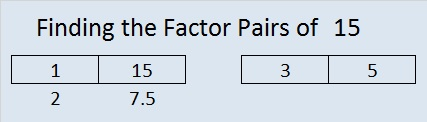When 15 is a clue in the FIND THE FACTORS 1 – 10 or 1 – 12 puzzles, use 3 and 5 as the factors.

If you added the first nine counting numbers together, what sum would you get? What is 1 + 2 +3 + 4+ 5 + 6 + 7 + 8 + 9?

Would you get the same answer by adding (1 + 9) + (2 + 8) + (3 +7) + (4 + 6) + 5?

These are two of the many fun questions you can explore when you try to make a magic square. What is a magic square? If you can place the numbers from 1 to 9 in the box below so that the sum of any row, column, or diagonal will equal the sum of any other row, column, or diagonal, then you will have made a 3 x 3 magic square. The sum of a row, column, or diagonal in a magic square is called the magic sum.Clearly it is not a magic square yet. In fact, only one of the numbers is positioned where it needs to be. Which number do you think is already in the correct position?

When it becomes a magic square, what will the magic sum be? One student noticed that in its current state the sums of the rows are 6, 15, and 24. The sums of the columns are 12, 15, 18. The sums of the diagonals are 15 and 15. Since 15 occurs most often, could the magic sum be 15? One way to determine what the magic sum should be is to add the sums of all three rows and then divide by the number of rows. Since 1 + 2 + 3 + 4 + 5 + 6 + 7 + 8 + 9 = 45 and 45 ÷ 3 = 15, then 15 is indeed the magic sum.

Here are a few easy-to-remember steps to construct a 3 x 3 magic square quickly.

Step 1: Draw a tic-tac-toe board and put 5 in the middle.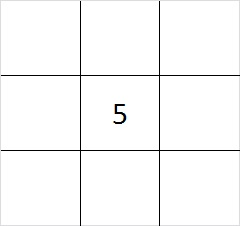Step 2: Put one of the even numbers in one of the corners.  You have four different choices, 2, 4, 6, or 8. The illustration is for the number 2, but any of the even numbers will work.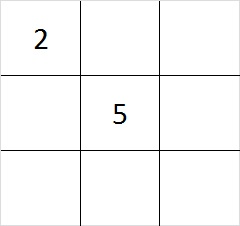Step 3: Subtract your even number from 10 to find its partner. 4 + 6 are partners and so are 2 + 8. Put the partner of the number you chose for step 1 in the corner that is diagonal to it.Step 4: Put the other two even numbers in the remaining corners. Yes, you have two choices where to put the numbers. Either choice will work.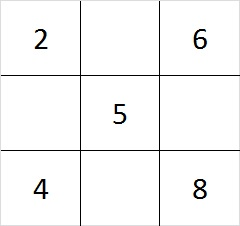Step 5: Since 6 + 8 = 14 and 15 – 14 = 1, put 1 in the cell between the 6 and the 8. Do similar addition and subtraction problems on each side of the square to determine where to place the 3, 7, and 9. You can work clockwise or counter clockwise, or skip around the square doing the addition and subtraction problems; it doesn’t matter.

This finished magic square looks like this: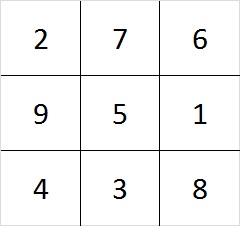Check it out! Every row, column, and diagonal adds up to 15!

As we created the square, we made choices. First we chose between 4 even numbers, and later we had 2 more choices. Notice that 4 x 2 = 8. There are 8 different ways to make a 3 x 3 magic square! (However, they are all really the same square turned upside down, rolled on its side, viewed from the back. etc.)

There are 880 different ways to make a 4 x 4 magic square. Look over the related articles at the end of this post to learn more about magic squares that are bigger than 3 x 3.

Speaking of magic squares, when I look at the square logic puzzle below, something magical happens. This puzzle has nine clues in it, and all of them are perfect squares. I can use those nine clues to construct a complete multiplication table. If you finish the same puzzle, your multiplication table will look exactly like mine because this puzzle has only one solution.The level 3 puzzle below is only a little bit more difficult. To solve it place the numbers 1 – 10 in the top row and again in the first column so that those placed numbers are the factors of the given clues. Again there is only one solution, and you will need to use logic to find it. Click 10 Factors 2014-01-06 for more puzzles and last week’s answers.May we all find a little bit more magic in our lives!